# Texas Go Math Grade 4 Lesson 10.2 Answer Key Divide Using Partial Quotients

Refer to our Texas Go Math Grade 4 Answer Key Pdf to score good marks in the exams. Test yourself by practicing the problems from Texas Go Math Grade 4 Lesson 10.2 Answer Key Divide Using Partial Quotients.

## Texas Go Math Grade 4 Lesson 10.2 Answer Key Divide Using Partial Quotients

Essential Question

How can you use partial quotients to divide by 1-digit divisors?

Unlock the Problem

At camp, there are 5 players on each lacrosse team. If there are 125 people on lacrosse teams, how many teams are there?

• Underline what you are asked to find.
• Circle what you need to use.
• What operation can you use to find the number of teams?

One Way Use partial quotients.

In the partial quotient method of dividing, multiples of the divisor are subtracted from the dividend and then the partial quotients are added together.

Divide. 125 ÷ 5 Write.STEP 1
Start by subtracting a greater multiple, such as 10 times the divisor. For example, you know that you can make at least 10 teams of 5 players.

Continue subtracting until the remaining number is less than the multiple, 50.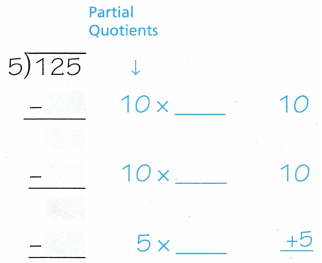On adding partial quotients : 20 + 5 = 25

Remainder : 0

STEP 2
Subtract smaller multiples, such as 5, 2, or 1 times the divisor until the remaining number is less than the divisor. In other words, keep going until you no longer have enough players to make a team.
Then add the partial quotients to find the quotient.
So, there are ____________ lacrosse teams.

So, there are 25 lacrosse teams.

Math Talk

Mathematical Processes
Explain how you found the total number of teams after finding the partial quotients.

We can find the total number of teams by adding the partial quotients.

Another Way Use area models to record the partial quotients.

Jawd and Mi also found the number of teams using partial quotients. They recorded the partial quotients using area models. they each still had 25 as the quotient.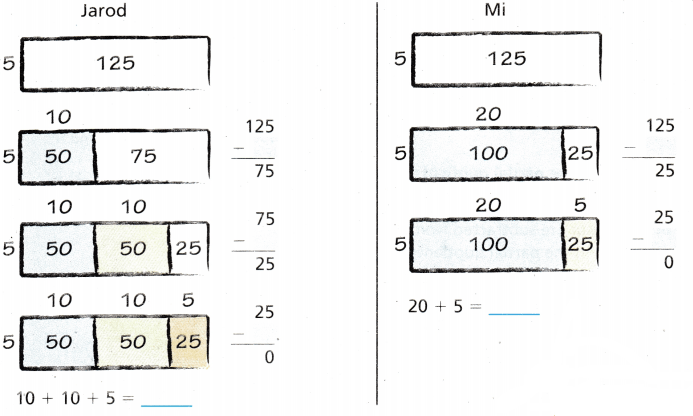Jarod : 10+10+5 = 25

Mi : 20+5 = 25

Math Talk

Mathematical Processes
Explain why you might prefer to use one method rather than the other.
Answer: We choose the partial quotient division method to complete the given problem in fewer steps.

Share and Show

Question 1.
Lacrosse is played on a field 330 ft long. How many yards long is a lacrosse held? (3 feet = 1 yard)
Divide. Use partial quotients.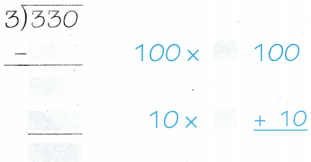So, the lacrosse field is _____ yards long.100 x 1 = 100

10 x 1 = 10

Total : 100 + 10 = 110

Divide. Use partial quotients.

Question 2.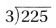On adding partial quotients = 70+5 = 75

Remainder = 0

Divide. Use area models to record the partial quotients.

Question 3.
428 ÷ 4 = __________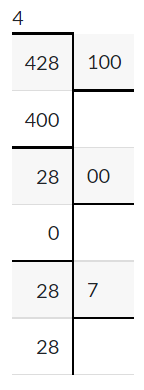On adding partial quotients = 100+7 = 107

Remainder = 0

Math Talk

Mathematical Processes
Explain how you could Problems 2 and 3 a different way.

We can solve the above problems in long division way :

2.Quotient : 75

Remainder : 0

3. 428 ÷ 4Quotient : 107

Remainder : 0

Problem Solving

Use the table for 4-5.Question 4.
Multi-Step Rob filled 9 plastic boxes with basketball cards and hockey cards. There were the same number of cards in each box. How many cards did he put iii each box?

Number of basket ball cards = 189

Number of hockey cards = 63

Total number = 189 + 63

=252

Now, Total number of plastic cards = 9

So, 252/9

= 28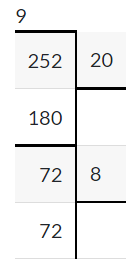On adding partial quotients = 20+8 = 28

Therefore, there are 28 cards in each box.

Remainder = 0

Question 5.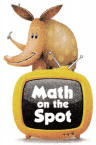H.O.T. Multi-Step Rob filled 3 fewer plastic boxes with football cards than the lime boxes he filled with basketball cards and hockey cards. How many boxes did he fill? How many football cards were in each box?

Question 6.
H.O.T. Communicate What is the least number you can divide by 5 to get a three-digit quotient? Explain how you found ‘our answer.

Question 7.
On the American frontier, salt was 4 times as expensive as beef Suppose a family on the frontier buys 112 pounds of beef. How much salt could they purchase with the same amount of money?
(A) 28 pounds
(B) 13 pounds
(C)22 pounds
(D) 33 pounds

Given that, salt was 4 times expensive as beef.

So, According to given condition

112 pounds of beef =Therefore, 28 pounds of salt they purchase with the same amount of money.

Question 8.
Multi-Step A family that owns an orchard collects 153 pounds of apples in one day and 126 pounds the next day. If the harvest is divided equally among 9 containers, how many pounds of apples are in each container?
(A) 19 pounds
(B) 37 pounds
(C) 31 pounds
(D) 27 pounds

The number of apples collected in one day = 153 pounds

The number of apples collected in next day = 126 pounds

Total = 153 + 126

= 279

Number of containers = 9

Now, 279/9 =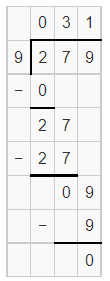Therefore, 31 pounds of apples are in each container

Question 9.
Apply Zachary’s family is planning a trip to Washington D.C. They will drive 837 miles from their home in St. Louis, Missouri. Zachary’s dad plans to make the trip in 3 days. How many miles should they plan to drive in each day if they want to drive the same distance every day?
(A) 314miles
(B) 239 miles
(C) 409miles
(D) 279 miles

The total distance Zachary’s family travels = 837 miles

Total number of days = 3

According to given condition,

837/3Therefore, 31 miles  they should plan to drive in each day if they want to drive the same distance every day.

TEXAS Test Prep

Question 10.
There are 80 fourth grade students and 46 fifth grade students who signed up to learn how to play lacrosse. If there are 6 students in each group, how many groups are there?
(A) 120
(B) 20
(C) 21
(D) 12

Number of forth grade students = 80

Number of fifth grade students = 46

Total = 126

Given, If there are 6 students un each group.

Now, 126/6

=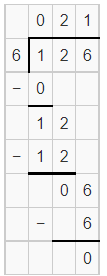Therefore, there are 21 groups.

### Texas Go Math Grade 4 Lesson 10.2 Homework and Practice Answer Key

Divide. Use partial quotients.

Question 1.On adding partial quotients = 90+0 = 90

Remainder = 0

Question 2.On adding partial quotients = 40+1 = 41

Remainder = 0

Question 3.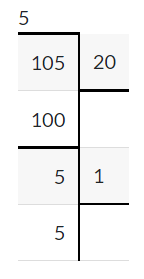On adding partial quotients = 20+1 = 21

Remainder = 0

Question 4.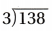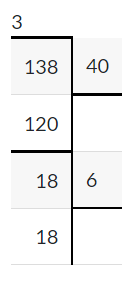On adding partial quotients = 40+6 = 46

Remainder = 0

Question 5.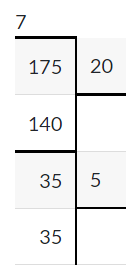On adding partial quotients = 20+5 = 25

Remainder = 0

Question 6.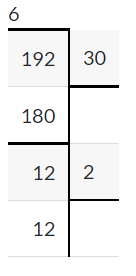On adding partial quotients = 30+2 = 32

Remainder = 0

Divide. Use area models to record the partial quotients.

Question 7.
104 ÷ 8 = _________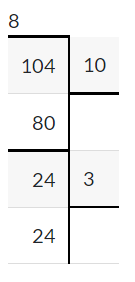On adding partial quotients = 10+3 = 13

Remainder = 0

Question 8.
210 ÷ 7 = _________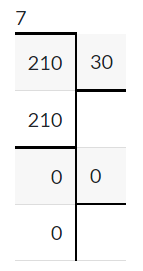On adding partial quotients = 30+0 = 30

Remainder = 0

Question 9.
365 ÷ 5 = _________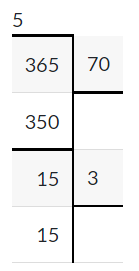On adding partial quotients = 70+3 = 73

Remainder = 0

Problem Solving

Question 10.
Valerie drove 320 miles in 5 hours. If she drove the same amount of miles each hour, how many miles did she drive in one hour?

Total distance = 320 miles

Total time = 5 hours

According to given condition, if she drove the same amount of miles each hour

Distance travels in 1 hour = 320/5

=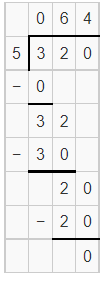Therefore, she drives 64 miles in each hour.

Question 11.
Keisha has 128 photos to put in a photo album. Each page holds 8 photos. How many pages will Keisha fill?

Total number of photos = 128

Number of photos each photo hold = 8

Now,Therefore, Keisha can fill 16 pages.

Lesson Check

Question 12.
Mr. Carson spent $144 on 8 bushes for his ‘yard. If each hush costs the same amount, how much did each bush cost? (A)$22
(B) $18 (C)$23
(D) $20 Answer: Number of bushes = 8 Total cost of 8 bushes =$144

If each hush costs the same amount,  Now, 144/8 =Therefore, each bush cost $18. Question 13. A furniture company moved 126 couches from their warehouse to the showroom. The truck made 9 trips to deliver the couches. If the truck carried the same number of couches in each trip, how many couches did the truck carry in one trip? (A) 13 (B) 18 (C) 15 (D) 14 Answer: The number of couches company moves to showroom = 126 The number of trips the truck made = 9 If the truck carried the same number of couches in each trip, NowTherefore, The truck carries 14 couches in one trip. Question 14. Leticia has$138 to spend on gifts for 6 friends. Each gift will cost the same amount of money. How much will she spend on each gift?
(A) $23 (B)$21
(C) $24 (D)$22

Total number of Leticia friends = 6

The amount she spent = $138 Also given, Each gift will cost the same amount of money. So,Therefore, Leticia spent$23 for each gift.

Question 15.
A custom order baseball cap company can make 320 caps in 5 days. If they make the same number of caps each day, how many caps can they make in one day?
(A) 50
(B) 80
(C) 64
(D) 70

The number of caps Baseball cap company make = 320

Number of days = 5

If they make the same number of caps each day,

Now,Therefore, they can make 64 caps in one day.

Question 16.
Multi-Step Marshall can buy 5 tee shirts for $60. If each shirt costs the same amount, what is the cost of 4 tee shirts? (A)$12
(B) $48 (C)$15
(D) $60 Answer: Number of tee shirts = 5 Total amount for 5 tee shirts =$60

If each shirt costs the same amount,

Now,The cost of 1 each shirt = $12 Now, the cost of 4 tee shirts =$12 x 4 = 48

Therefore, the cost 4 tee shirts = $48. Question 17. Multi-Step The Jones family paid$36 for 2 burger meals and 4 chicken strip meals. Each of the meals costs the same amount of money. What would the Jones family have paid if they bought only the 4 chicken strip meals?
(A) $24 (B)$18
(C) $12 (D)$6
The amount Jones family paid for 2 burger and 4 chicken strips = $36 Given, Each of the meals costs the same amount of money.$36/6 = $6 So, each meal cost$6 each.
4 x $6 =$24.